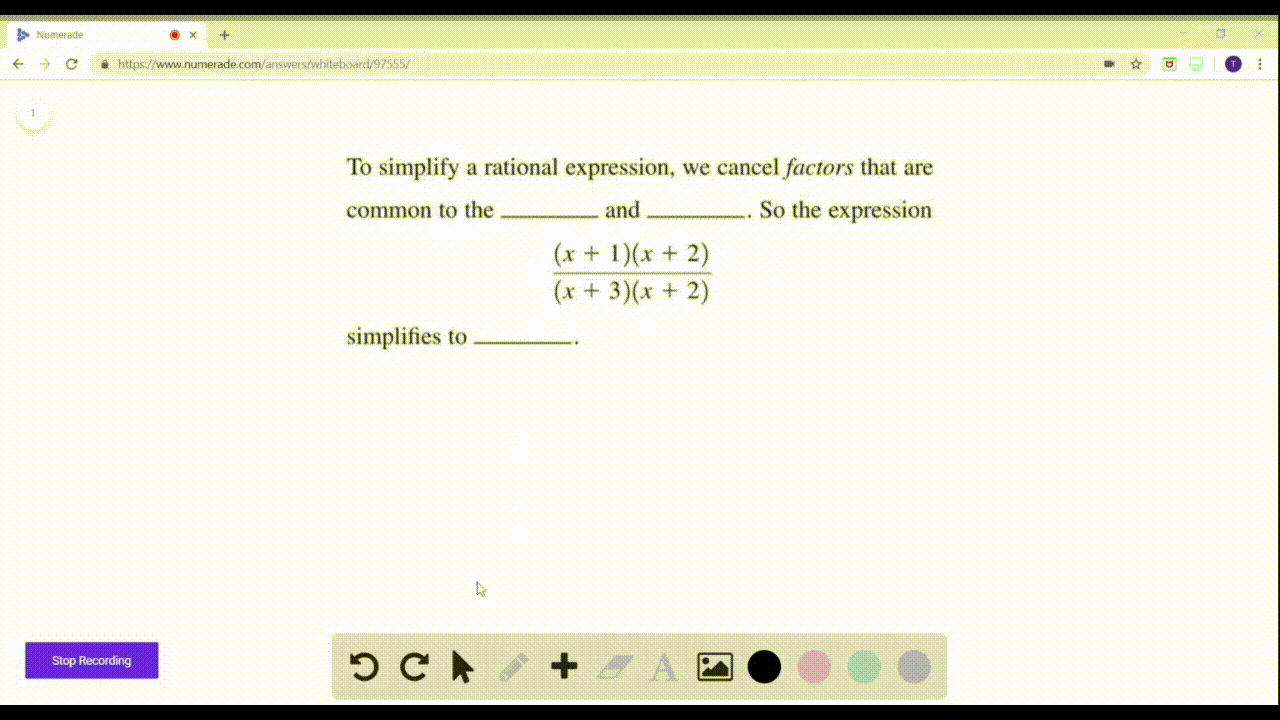Enroll in one of our FREE online STEM summer camps. Space is limited so join now!View Summer Courses### To simplify a rational expression, we cancel fact…

01:33Winsor School

Need more help? Fill out this quick form to get professional live tutoring.

Get live tutoring
Problem 1

Which of the following are rational expressions?
$${ (a) } \frac{3 x}{x^{2}-1} \quad \text { (b) } \frac{\sqrt{x+1}}{2 x+3} \quad \text { (c) } \frac{x\left(x^{2}-1\right)}{x+3}$$

choices (a) and (c)

## Discussion

You must be signed in to discuss.

## Video Transcript

so I asked if I which of these three electoral expressions so irrational expression is when we have a polynomial divided by a pollen oatmeal cookie? Look at a three X is a polynomial. An X squared minus. One is also polynomial So a works. I'll be like a B is a screw of experts. Wanna pollen? No, because of this coat here. So this is not irrational expression. Then when we look at sea, you have X times X squared minus one which is equal to execute my sex. So yes, it's an opponent and ex minister is also a polynomial. So which means that see the devotional expression so into the question Look A and C irrational expect